Longest Common Subsequence

# Longest Common Subsequence

#### In this tutorial, you will learn how the longest common subsequence is found. Also, you will find working examples of the longest common subsequence in C, C++, Java and Python.

The longest common subsequence (LCS) is defined as the longest subsequence that is common to all the given sequences, provided that the elements of the subsequence are not required to occupy consecutive positions within the original sequences.

If S1 and S2 are the two given sequences then, Z is the common subsequence of S1 and S2 if Z is a subsequence of both S1 and S2. Furthermore, Z must be a strictly increasing sequence of the indices of both S1 and S2.

In a strictly increasing sequence, the indices of the elements chosen from the original sequences must be in ascending order in Z.
If

`S1 = {B, C, D, A, A, C, D}`

Then, `{A, D, B}` cannot be a subsequence of S1 as the order of the elements is not the same (ie. not strictly increasing sequence).

Let us understand LCS with an example.

If

```S1 = {B, C, D, A, A, C, D}
S2 = {A, C, D, B, A, C}```

Then, common subsequences are `{B, C}, {C, D, A, C}, {D, A, C}, {A, A, C}, {A, C}, {C, D}, ... `

Among these subsequences, `{C, D, A, C}` is the longest common subsequence. We are going to find this longest common subsequence using dynamic programming.

Before proceeding further, if you do not already know about dynamic programming, please go through dynamic programming.

## Using Dynamic Programming to find the LCS

Let us take two sequences:The following steps are followed for finding the longest common subsequence.

1. Create a table of dimension `n+1*m+1` where n and m are the lengths of X and Y respectively. The first row and the first column are filled with zeros.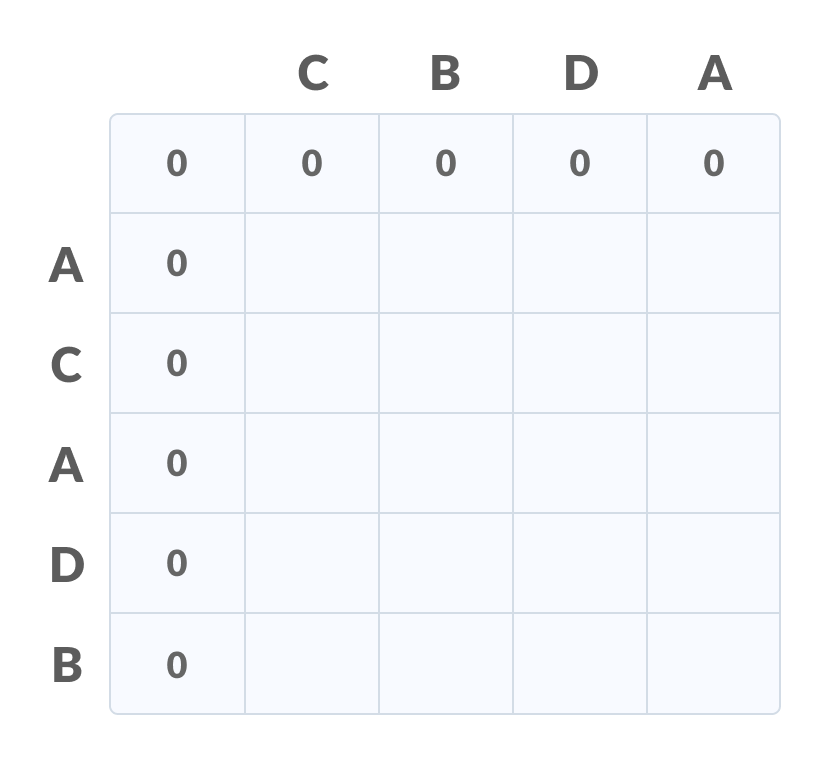2. Fill each cell of the table using the following logic.
3. If the character correspoding to the current row and current column are matching, then fill the current cell by adding one to the diagonal element. Point an arrow to the diagonal cell.
4. Else take the maximum value from the previous column and previous row element for filling the current cell. Point an arrow to the cell with maximum value. If they are equal, point to any of them.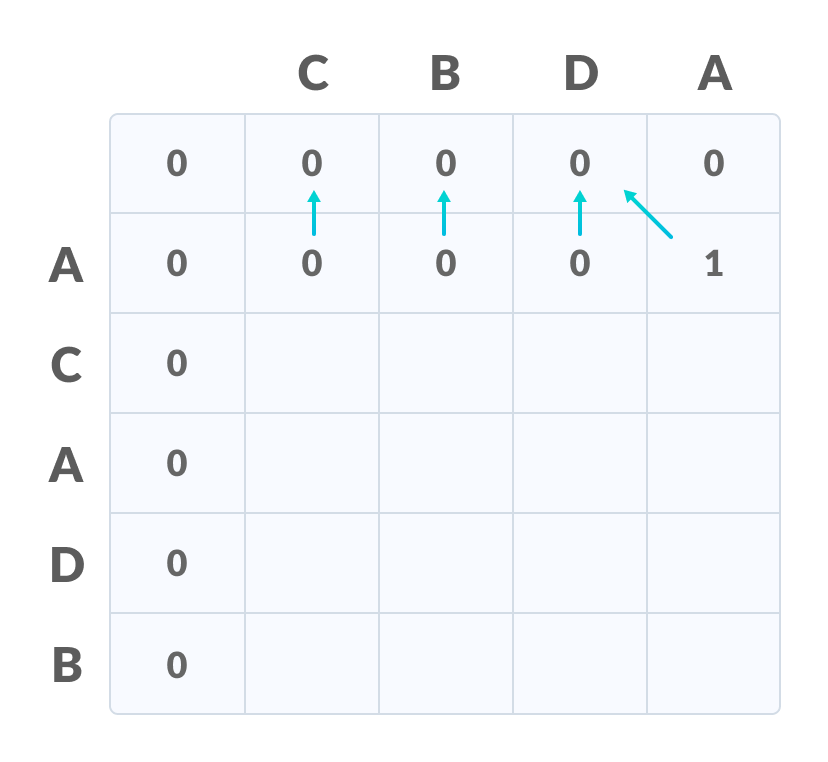5. Step 2 is repeated until the table is filled.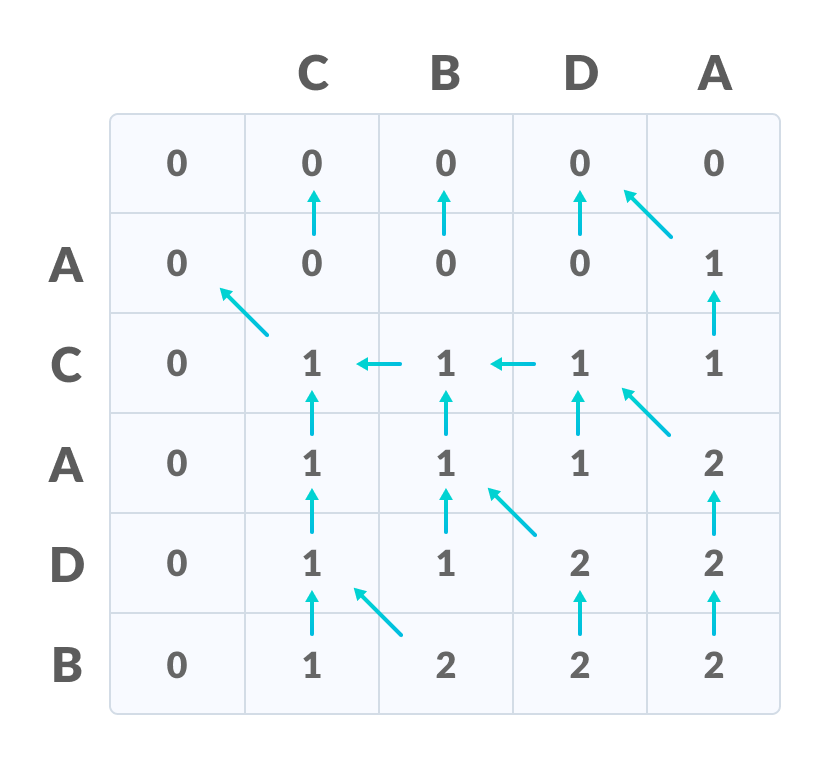6. The value in the last row and the last column is the length of the longest common subsequence.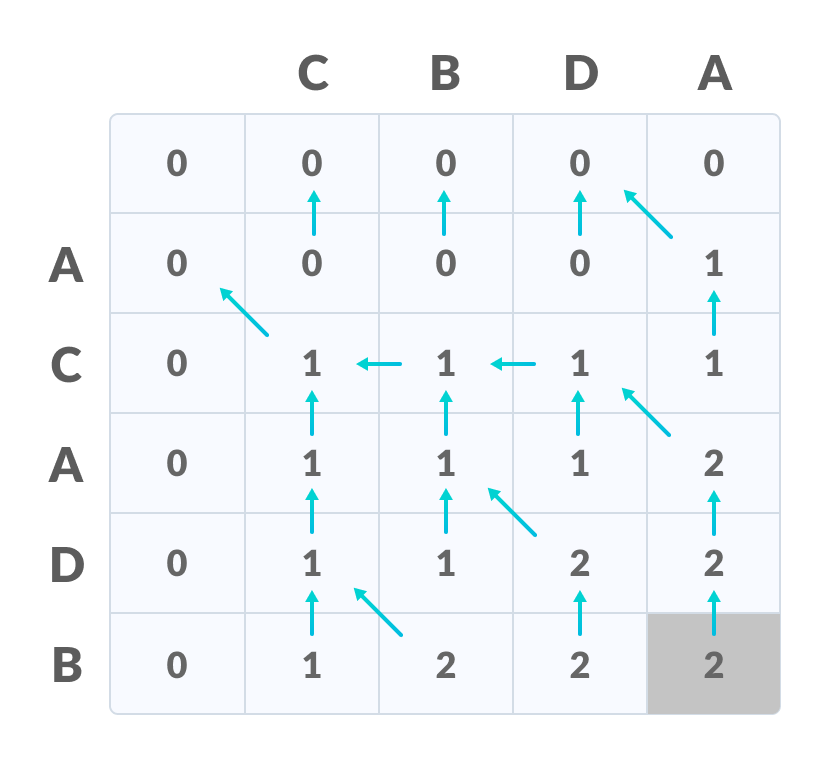7. In order to find the longest common subsequence, start from the last element and follow the direction of the arrow. The elements corresponding to () symbol form the longest common subsequence.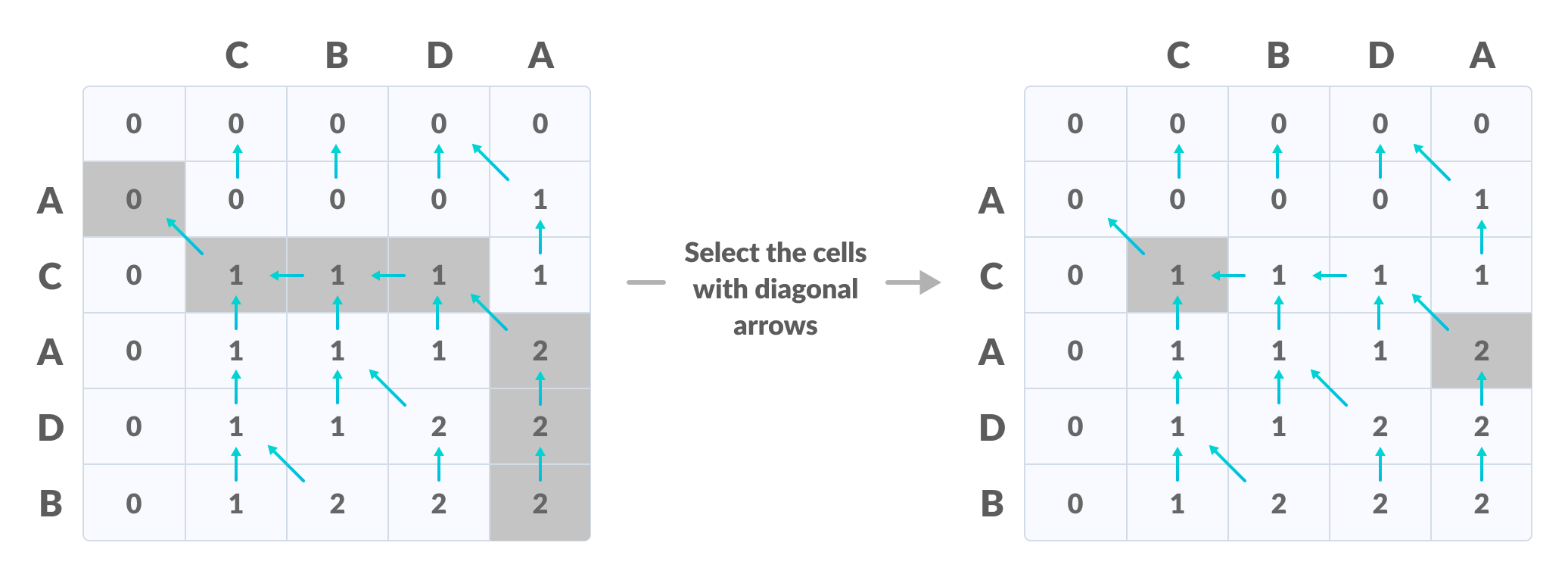Thus, the longest common subsequence is CD.How dynamic programming algorithm is more efficient than the recursive algorithm while solving an LCS problem?

The method of dynamic programming reduces the number of function calls. It stores the result of each function call so that it can be used in future calls without the need for redundant calls.

In the above dynamic algorithm, the results obtained from each comparison between elements of X and the elements of Y are stored in a table so that they can be used in future computations.

So, the time taken by a dynamic approach is the time taken to fill the table (ie. O(mn)). Whereas, recursion algorithm has the complexity of 2max(m, n).

## Longest Common Subsequence Algorithm

``````X and Y be two given sequences
Initialize a table LCS of dimension X.length * Y.length
X.label = X
Y.label = Y
LCS[] = 0
LCS[] = 0
Start from LCS
Compare X[i] and Y[j]
If X[i] = Y[j]
LCS[i][j] = 1 + LCS[i-1, j-1]
Point an arrow to LCS[i][j]
Else
LCS[i][j] = max(LCS[i-1][j], LCS[i][j-1])
Point an arrow to max(LCS[i-1][j], LCS[i][j-1])
``````

## Python, Java and C/C++ Examples

``````# The longest common subsequence in Python

def lcs(S1, S2, m, n):
L = [[0 for x in range(n+1)] for x in range(m+1)]

for i in range(m+1):
for j in range(n+1):
if i == 0 or j == 0:
L[i][j] = 0
elif S1[i-1] == S2[j-1]:
L[i][j] = L[i-1][j-1] + 1
else:
L[i][j] = max(L[i-1][j], L[i][j-1])

index = L[m][n]

lcs = [""] * (index+1)
lcs[index] = ""

i = m
j = n
while i > 0 and j > 0:

if S1[i-1] == S2[j-1]:
lcs[index-1] = S1[i-1]
i -= 1
j -= 1
index -= 1

elif L[i-1][j] > L[i][j-1]:
i -= 1
else:
j -= 1

print("S1 : " + S1 + "\nS2 : " + S2)
print("LCS: " + "".join(lcs))

S2 = "CBDA"
m = len(S1)
n = len(S2)
lcs(S1, S2, m, n)
``````
``````// The longest common subsequence in Java

import java.io.*;

class LCS {
static void lcs(String S1, String S2, int m, int n) {
int[][] LCS_table = new int[m + 1][n + 1];

for (int i = 0; i <= m; i++) {
for (int j = 0; j <= n; j++) {
if (i == 0 || j == 0)
LCS_table[i][j] = 0;
else if (S1.charAt(i - 1) == S2.charAt(j - 1))
LCS_table[i][j] = LCS_table[i - 1][j - 1] + 1;
else
LCS_table[i][j] = Math.max(LCS_table[i - 1][j], LCS_table[i][j - 1]);
}
}

int index = LCS_table[m][n];
int temp = index;

char[] lcs = new char[index + 1];
lcs[index] = '\0';

int i = m, j = n;
while (i > 0 && j > 0) {
if (S1.charAt(i - 1) == S2.charAt(j - 1)) {
lcs[index - 1] = S1.charAt(i - 1);

i--;
j--;
index--;
}

else if (LCS_table[i - 1][j] > LCS_table[i][j - 1])
i--;
else
j--;
}

System.out.print("S1 : " + S1 + "\nS2 : " + S2 + "\nLCS: ");
for (int k = 0; k <= temp; k++)
System.out.print(lcs[k]);
System.out.println("");
}

public static void main(String[] args) {
String S2 = "CBDA";
int m = S1.length();
int n = S2.length();
lcs(S1, S2, m, n);
}
}
``````
``````// The longest common subsequence in C

#include <stdio.h>
#include <string.h>

int i, j, m, n, LCS_table;
char S1 = "ACADB", S2 = "CBDA", b;

void lcs()
{
m = strlen(S1);
n = strlen(S2);
for (i = 0; i <= m; i++)
LCS_table[i] = 0;
for (i = 0; i <= n; i++)
LCS_table[i] = 0;

for (i = 1; i <= m; i++)
for (j = 1; j <= n; j++)
{
if (S1[i - 1] == S2[j - 1])
{
LCS_table[i][j] = LCS_table[i - 1][j - 1] + 1;
}
else if (LCS_table[i - 1][j] >= LCS_table[i][j - 1])
{
LCS_table[i][j] = LCS_table[i - 1][j];
}
else
{
LCS_table[i][j] = LCS_table[i][j - 1];
}
}

int index = LCS_table[m][n];
char lcs[index + 1];
lcs[index] = '\0';

int i = m, j = n;
while (i > 0 && j > 0)
{
if (S1[i - 1] == S2[j - 1])
{
lcs[index - 1] = S1[i - 1];
i--;
j--;
index--;
}

else if (LCS_table[i - 1][j] > LCS_table[i][j - 1])
i--;
else
j--;
}

printf("S1 : %s \nS2 : %s \n", S1, S2);
printf("LCS: %s", lcs);
}

int main()
{
lcs();
printf("\n");
}``````
``````// The longest common subsequence in C++

#include <iostream>
using namespace std;

void lcs(char *S1, char *S2, int m, int n)
{
int LCS_table[m + 1][n + 1];

for (int i = 0; i <= m; i++)
{
for (int j = 0; j <= n; j++)
{
if (i == 0 || j == 0)
LCS_table[i][j] = 0;
else if (S1[i - 1] == S2[j - 1])
LCS_table[i][j] = LCS_table[i - 1][j - 1] + 1;
else
LCS_table[i][j] = max(LCS_table[i - 1][j], LCS_table[i][j - 1]);
}
}

int index = LCS_table[m][n];
char lcs[index + 1];
lcs[index] = '\0';

int i = m, j = n;
while (i > 0 && j > 0)
{
if (S1[i - 1] == S2[j - 1])
{
lcs[index - 1] = S1[i - 1];
i--;
j--;
index--;
}

else if (LCS_table[i - 1][j] > LCS_table[i][j - 1])
i--;
else
j--;
}

cout << "S1 : " << S1 << "\nS2 : " << S2 << "\nLCS: " << lcs << "\n";
}

int main()
{